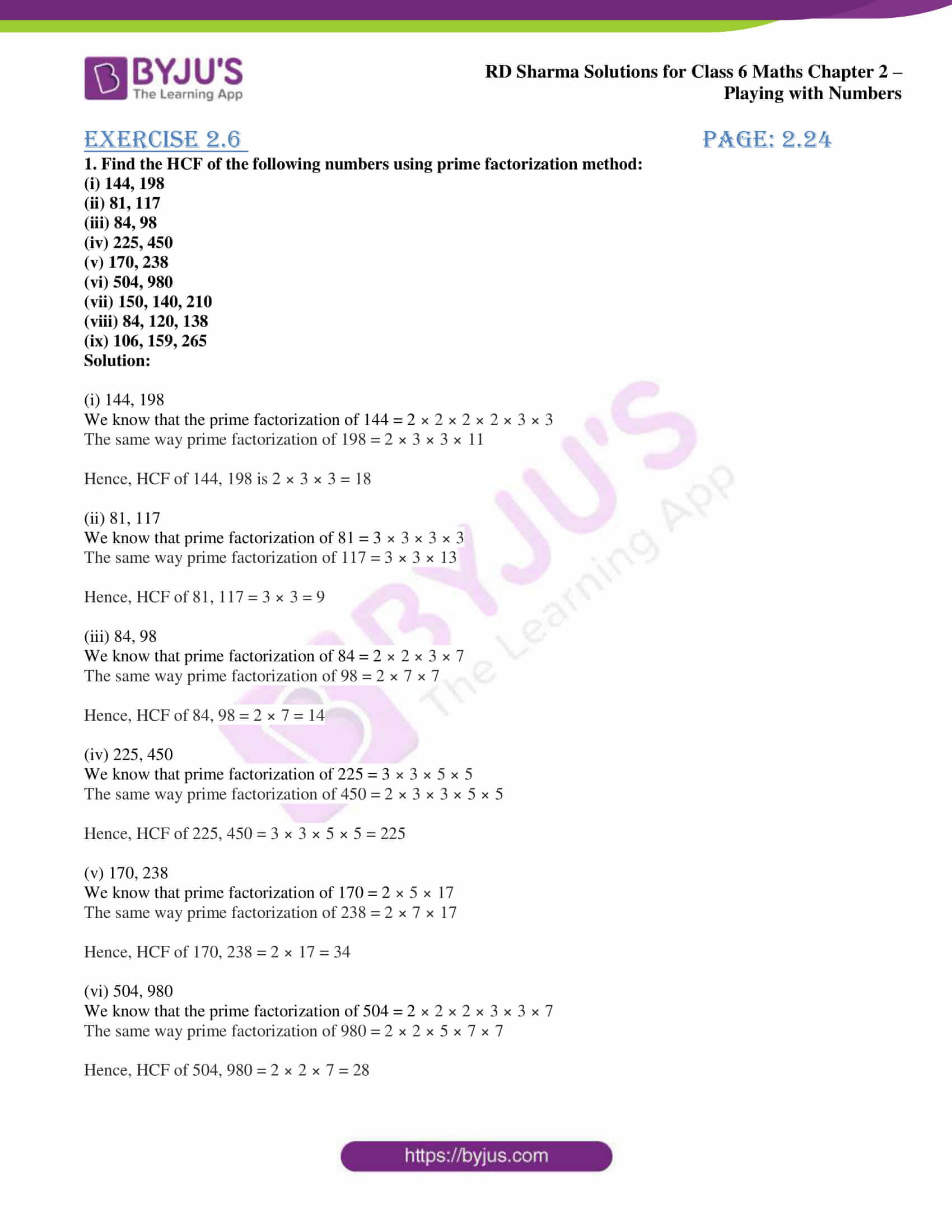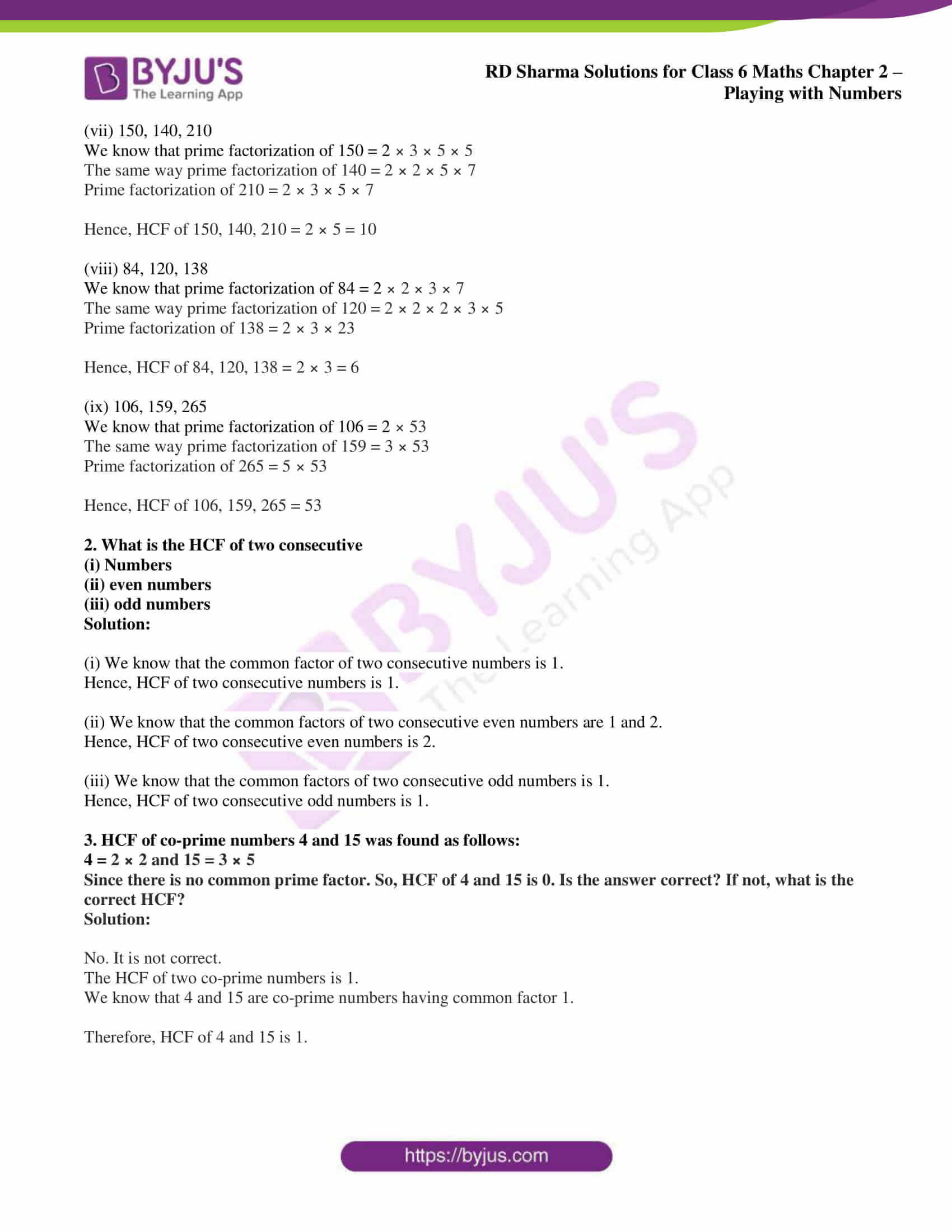# RD Sharma Solutions for Class 6 Maths Chapter 2: Playing with Numbers Exercise 2.6

## RD Sharma Solutions for Class 6 Maths Exercise 2.6 PDF

RD Sharma Solutions created by faculty at BYJU’S help students understand the basic concepts which are covered in each exercise. The aim of providing solutions is to improve confidence among students to appear for the exam. The solutions are provided in a comprehensive manner in a simple language based on the understanding capacity of students. The solutions PDF can be downloaded by the students while solving the exercise wise problems of RD Sharma textbook. RD Sharma Solutions for Class 6 Maths Chapter 2 Playing with Numbers Exercise 2.6 are provided here.

## RD Sharma Solutions for Class 6 Maths Chapter 2: Playing with Numbers Exercise 2.6 Download PDF## Access answers to Maths RD Sharma Solutions for Class 6 Chapter 2: Playing with Numbers Exercise 2.6

1. Find the HCF of the following numbers using prime factorization method:

(i) 144, 198

(ii) 81, 117

(iii) 84, 98

(iv) 225, 450

(v) 170, 238

(vi) 504, 980

(vii) 150, 140, 210

(viii) 84, 120, 138

(ix) 106, 159, 265

Solution:

(i) 144, 198

We know that the prime factorization of 144 = 2 × 2 × 2 × 2 × 3 × 3

The same way prime factorization of 198 = 2 × 3 × 3 × 11

Hence, HCF of 144, 198 is 2 × 3 × 3 = 18

(ii) 81, 117

We know that prime factorization of 81 = 3 × 3 × 3 × 3

The same way prime factorization of 117 = 3 × 3 × 13

Hence, HCF of 81, 117 = 3 × 3 = 9

(iii) 84, 98

We know that prime factorization of 84 = 2 × 2 × 3 × 7

The same way prime factorization of 98 = 2 × 7 × 7

Hence, HCF of 84, 98 = 2 × 7 = 14

(iv) 225, 450

We know that prime factorization of 225 = 3 × 3 × 5 × 5

The same way prime factorization of 450 = 2 × 3 × 3 × 5 × 5

Hence, HCF of 225, 450 = 3 × 3 × 5 × 5 = 225

(v) 170, 238

We know that prime factorization of 170 = 2 × 5 × 17

The same way prime factorization of 238 = 2 × 7 × 17

Hence, HCF of 170, 238 = 2 × 17 = 34

(vi) 504, 980

We know that the prime factorization of 504 = 2 × 2 × 2 × 3 × 3 × 7

The same way prime factorization of 980 = 2 × 2 × 5 × 7 × 7

Hence, HCF of 504, 980 = 2 × 2 × 7 = 28

(vii) 150, 140, 210

We know that prime factorization of 150 = 2 × 3 × 5 × 5

The same way prime factorization of 140 = 2 × 2 × 5 × 7

Prime factorization of 210 = 2 × 3 × 5 × 7

Hence, HCF of 150, 140, 210 = 2 × 5 = 10

(viii) 84, 120, 138

We know that prime factorization of 84 = 2 × 2 × 3 × 7

The same way prime factorization of 120 = 2 × 2 × 2 × 3 × 5

Prime factorization of 138 = 2 × 3 × 23

Hence, HCF of 84, 120, 138 = 2 × 3 = 6

(ix) 106, 159, 265

We know that prime factorization of 106 = 2 × 53

The same way prime factorization of 159 = 3 × 53

Prime factorization of 265 = 5 × 53

Hence, HCF of 106, 159, 265 = 53

2. What is the HCF of two consecutive

(i) Numbers

(ii) even numbers

(iii) odd numbers

Solution:

(i) We know that the common factor of two consecutive numbers is 1.

Hence, HCF of two consecutive numbers is 1.

(ii) We know that the common factors of two consecutive even numbers are 1 and 2.

Hence, HCF of two consecutive even numbers is 2.

(iii) We know that the common factors of two consecutive odd numbers is 1.

Hence, HCF of two consecutive odd numbers is 1.

3. HCF of co-prime numbers 4 and 15 was found as follows:

4 = 2 × 2 and 15 = 3 × 5

Since there is no common prime factor. So, HCF of 4 and 15 is 0. Is the answer correct? If not, what is the correct HCF?

Solution:

No. It is not correct.

The HCF of two co-prime numbers is 1.

We know that 4 and 15 are co-prime numbers having common factor 1.

Therefore, HCF of 4 and 15 is 1.

### RD Sharma Solutions for Class 6 Maths Chapter 2 – Playing with Numbers Exercise 2.6

RD Sharma Solutions Class 6 Maths Chapter 2 Playing with Numbers Exercise 2.6 help students to understand the method of finding HCF and prime factorization which can be used to determine the HCF.

### Key features of RD Sharma Solutions for Class 6 Maths Chapter 2: Playing with Numbers Exercise 2.6

• RD Sharma Solutions created by subject matter experts help students get an overall understanding of the concepts.
• Solving ample number of examples and exercise wise problems makes the students familiar with the type of questions that would appear in the exam.
• The students by solving exercise wise problems improve their confidence to appear for the exam.
• It helps students to answer the problems more accurately and efficiently with good speed.